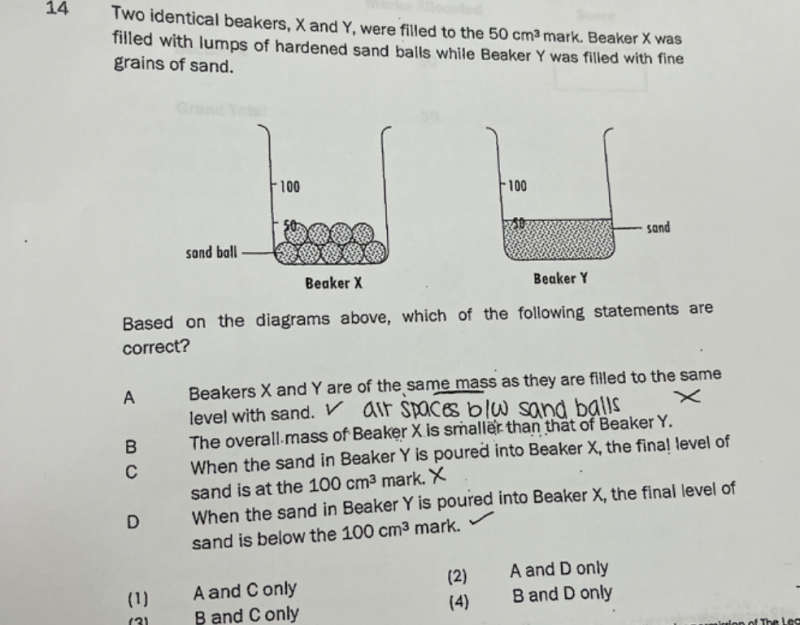# QuestionHi, any idea why answer is (4)?

Mass = Volume  x Density     meaning the increase in mass depends on the increase in volume and density.

From the picture, Beaker X has gaps on the sides and in between the balls of sand, so it is not as densely packed as Beaker Y.

As for the volume, since it did not go above 50cm, it cannot be greater than Beaker Y.

So likely the mass in Beaker X can be smaller or equal than Beaker Y.

That makes (B) valid.

(D) is true because the amount of sand in Beaker X is not all filled at 50cm3 mark, there are still gaps in the beaker . Due to these gaps, so when Beaker Y’s sand is poured in , the sand will fill up the gaps first, therefore it will be less than 100cm3 .

Hence answer (B) and (D) are possible making (4) the answer.

0 Replies 1 Like